﻿ Analysis of Grades 7 and 8 Physics Textbooks: A Quantitative Approach

### Analysis of Grades 7 and 8 Physics Textbooks: A Quantitative ApproachOPEN ACCESSPEER-REVIEWED

## Analysis of Grades 7 and 8 Physics Textbooks: A Quantitative Approach

Zemenu Mihret ZewdieDebre Markos College of Teacher Education, Debre Markos, Ethiopia

### Abstract

The purpose of this paper was to analyze grade 7 and 8 physics student textbooks for Ethiopian schools. The study was conducted based on the comments collected from the school teachers during physics handbook familiarization workshops. In addition, the Ministry of Education demands that textbooks currently in use as well as those that will be produced in the future will be greatly improved or revised. The main method of the research employed was content analysis. The different categories of grade 7 and 8 physics textbooks such as learning objectives, activities, figures and diagrams, text narratives, unit summaries, and end-of-unit exercises were sampled. Quantitative analysis techniques were employed and index values for students’ involvement were calculated. In addition, open-ended questionnaires were prepared, distributed, and collected from teachers and students to triangulate the data obtained from the six sample aspects of the textbooks. The data obtained from the textbooks were analyzed quantitatively and interpreted based on the index value interpretation guideline adapted from literature. The data obtained from the questionnaires were analyzed qualitatively and used to support the quantitative data. The results revealed that learning objectives were stated in all the units in the textbooks. In addition, the figures, diagrams, other drawings, and points that demand emphasis were put in attractive colors that grasp students for reading. However, the other aspects of the textbooks were found to have limitations. These aspects of the textbooks emphasized on memorization of facts, explanations, principles; the activities had immediate answers in the textbooks; figures and diagrams concentrated mainly for illustrative purpose; review questions and problems demanded simple memory and mere mathematical calculations based on previous learning of formulas; summaries reflected main points in the text narratives and limited to raise new questions and up-to-date scientific researches. Accordingly, conclusions were drawn and recommendations for further improvements and revisions were forwarded.

• Zewdie, Zemenu Mihret. "Analysis of Grades 7 and 8 Physics Textbooks: A Quantitative Approach." American Journal of Educational Research 2.1 (2014): 44-49.
• Zewdie, Z. M. (2014). Analysis of Grades 7 and 8 Physics Textbooks: A Quantitative Approach. American Journal of Educational Research, 2(1), 44-49.
• Zewdie, Zemenu Mihret. "Analysis of Grades 7 and 8 Physics Textbooks: A Quantitative Approach." American Journal of Educational Research 2, no. 1 (2014): 44-49.

 Import into BibTeX Import into EndNote Import into RefMan Import into RefWorks

### 1. Introduction

Physics is defined as the study of the nature of matter, energy, and their interactions. It is defined as a science of observation and measurement that enables us to understand the world at large and the laws of nature. Physics is one of the natural science subjects given as a linear subject starting from grade 7 to 12 in Ethiopian schools. The Federal Democratic Republic of Ethiopia Ministry of Education (2009a) pointed out that the learning of physics enables students/learners to understand the physical world, to carryout observations and experiments related with physical events and phenomena, enhance interest in nature. The known physics educator Redish (2002) has also suggested that students going to study other sciences need to understand physics as part of their scientific education. In learning physics, students gain scientific knowledge, skills, and attitudes which enable them realize that problems can be solved. That is, it develops self confidence in the part of learners not only to solve problems in physics but also other real-life problems. The grade 7 physics textbook for Ethiopian schools consisted of 8 units: Physics and Measurement, Motion, Force and Newton’s laws of Motion, Work, Energy, & Power, Simple Machines, Temperature & Heat, Sound, and Electricity & Magnetism. The grade 8 physics textbook for Ethiopian schools consisted of 6 units: Physics and Measurement, Motion in One Dimension, Pressure, Heat Energy, Electricity & Magnetism, and Light.

Furthermore, studying physics can have the following advantages: helps to understand the working principles of many of our daily utensils and tools, helps to discover nature and how it works, helps to apply the knowledge of physics to other sciences and disciplines, and helps to solve practical and real-life problems (MoE, 2011a). Having such essential role to understand nature and natural phenomena, physics should be taught by doing or practical activities rather than telling facts to students. Following the new Education and Training Policy (ETP), a new national wide curriculum development and implementation program for all grade levels was undertaken in which science and mathematics curricula were the most prioritized subjects in the national curriculum (TGE, 1994). In this curriculum framework, it is clearly indicated that it is the learner-centered approaches and the constructivist epistemology that the teaching-learning process should follow. In this approach, physics as a process is advocated rather than physics as a product or body of established knowledge. This is because students are engaged in knowledge building; there are other dimensions also that they come up with in addition to knowledge.

The Federal Democratic Republic of Ethiopia Ministry of Education has undertaken science and mathematics subjects the most prioritized so that strategies for improving science and mathematics education has been prepared (MoE, 2009b). This document emphasized the importance of quality teaching and learning materials to bring quality in science and mathematics education. Students’ textbooks, students’ workbooks, teacher’s guide, teacher’s handbook, syllabus, minimum learning competency guides, audio-video materials, and other teaching learning resources are taken to be important in improving science and mathematics education.

Textbooks as curriculum materials play very important role in serving as supplementary materials by incorporating explanation of terms and the findings of many different researches. In Ethiopian context where there are no enough curriculum materials, textbooks are taken to be the basic sources of ideas and information. Each student will have a textbook to take home and use it free of cost and will get it back at the end of the academic year. According to the Amhara National Regional State Education Bureau (2001), textbooks are the most useful means of communicating knowledge and they are the first and basic input materials in Ethiopian schools. Many other literatures concerning the role of textbooks pointed out that quality textbooks are very important for both students and teachers as they determine the contents to be taught and the way these contents to be taught (Dalim and Mubarrak, 2013; Mergo, 2012; MoE, 2002; Bhatti and Hashmi, n.d).

Like other subjects, science and mathematics teaching has moved from teacher-centered to student-centered approach. This in turn has led to a change in the role of science and mathematics textbooks. According to Dalim and Mubarrak (2013), a textbook is considered as good if it is more student-centered, promoting self-directed learning, allowing students to learn by themselves at their own pace and have activities for students to enhance the mastery of the topic. McKeachie in National Academy of Sciences (1997) indicated that, for many people, visual processing (i.e., reading) is faster than auditory processing (i.e., listening to lectures), making textbooks a very effective resource. A textbook, an educational input, can bring a difference in educational equity and quality (Laws and Horsley, 2006) and serves as a catalyst to discussion or instruction, serve as references for students, provide background information, or supply examples and applications of key ideas in science (Haury and Rillero, 1994). Especially, Haury and Rillero (1994) emphasized that textbooks should be prepared in a way to engage students for learning.

In order to assure whether textbooks meet the necessary requirements or whether they engage students for learning or whether they are good for active learning, they must be evaluated. The quality of the textbooks should be assessed and evaluated during implementation by outside consultants and who were not part of the textbook development and selection process (MoE, 2009b). Many scholars indicated that textbooks are being used as a major source of information in teaching a particular subject, the quality and accuracy of the content is crucial for their educational effectiveness (Dalim and Mubarrak, 2013; Khine, 2008). Apart from simple eye-boring examination of grade 7 and 8 physics textbooks, the writer of this paper collected information from in-service physics teachers, pre-service student physics teachers, and physics teachers during physics handbook familiarization workshops which helped to identify the presence of the problem. Moreover, MoE (2002) and TGE (1994) declared the demand of the new education and training policy directions to make improvements and revisions in textbooks to meet national and international standards. Consequently, the present study was designed to analyze the different aspects of grade 7 and 8 physics textbooks such as learning objectives, text narratives, figures and diagrams, activities, exercises at the end of the units, and summaries.

### 2. Conceptual Framework of This Study: Quantitative Analysis of Textbooks

Different techniques could be employed to analyze textbooks. Selecting an appropriate technique of analyzing textbooks is at our disposal. Bloom’s taxonomy, the analysis of conceptual development, analysis of the inquiry level of textbooks, quantitative analysis of different aspects of textbooks, etc are some of the techniques to analyze textbooks (Engida, 2005). In this paper, quantitative analysis of different aspects of grade 7 and 8 physics textbooks were investigated. These aspects of the textbook can be arranged into six categories: Learning objectives, Text narratives, Activities, Figures and Diagrams, Unit summaries, and Exercises at the end of units. Each category can be again broken down into points to identify and calculate the index for students’ involvement (Dalim and Mubarrak, 2013; Mergo, 2012; Engida, 2005).

2.1. Learning Objectives

Textbooks should explain the learning objectives before the learning process takes place (Dalim and Mubarrak, 2013). To analyze the learning objectives, distribution of objectives in the textbooks can be seen. On the other hand, to determine the index of learning objectives of a textbook, the total number of units with objectives are counted and divided by the total number of units with the textbook. That is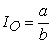Where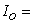index of learning objectives; a= total number of units with objectives; b= total number of units

2.2. Text Narratives

Text narratives are one of several aspects of textbooks. In analyzing science textbooks, different scholars have used text narratives as one category (Dalim and Mubarrak, 2013; Mergo, 2012; Engida, 2005). The criteria to calculate the index of text narratives are listed below:

a= statements of facts given by the author

b= stated conclusions or generalizations about meaning or relationships between terms

c= definitions of concepts or principles

e= questions involving the students to analyze some data

f= statements requiring the students to formulate and draw their own conclusions

g= statements telling the students to solve a problem; perform an activity

h= questions that are asked to arouse student interest and no immediate answer to the questions in the text

i= statements leading the students to look at a figure or diagram, procedural instructions in activities, and statements not fitting any of the above lists

j= rhetoric questions

From the above 10 lists concerning text narratives, i and j have no any contribution to the analysis of text narratives in the textbooks and can be eliminated from the calculation. Thus,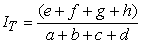In the above formula, lists a, b, c, and d are related to passive student learning whereas lists e, f, g, and h are considered as active student learning. Points i and j have no contribution to quality and usefulness of textbooks (Dalim and Mubarrak, 2013; Mergo, 2012; Engida, 2005).

2.3. Activities

According to Robert (1962), textbooks should have several purposeful activities that require students to work together. The following formula is used for student involvement of activities: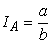Where a=number of pages that have activities and b=total number of pages

2.4. Figures and Diagrams

Learning will be facilitated when the lesson is presented in the visual form using figures, diagrams, pictures, graphs, illustrations, and photographs (Yimulaw, 2007). The following formula is used to calculate index of student involvement for figures and diagrams: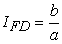Where a=figures and diagrams are used for illustrative purpose,

b= figures and diagrams requiring students to analyze data or perform some activity

If the diagram or figure is used to illustrate how to set up the apparatus for some activity or if it does not fit in any of the lists above, it does not have any contribution for index of student involvement for figures and diagrams.

2.4. End – of - unit Exercises (Review Questions and Problems)

According to scholars in the area, the following four lists are used to calculate index of student involvement for end of unit exercises (Dalim and Mubarrak, 2013; Mergo, 2012; Engida, 2005).

These are:-

a= questions that have answers directly from the textbook

c= questions requiring the students to apply what they have learned to new situations

d= questions requiring students for problem solving

Thus,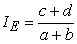2.5. Summaries at the End of Units

Summaries are very important in providing students with new questions that lead for further learning after completing a unit or acquitting students with current issues and researches in science and technology. The following formula is used to calculate the index of student involvement Is for summaries at the end of units: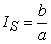Where a=summaries that repeat the conclusions of the unit and summarizes the same idea from the texts.

b= summaries that raise new questions, the answers to which are not available in the text or are subjects of current research in science

2.6. Index Value Interpretation

Dalim and Mubarrak (2013) pointed out that the index of student involvement values indicate how good the textbook is in promoting the students’ engagement in active learning. The guideline for index values interpretation is given in Table 1 below.

### 3. Research Methodology and Procedures

This research paper attempted to analyze grade 7 and 8 physics textbooks. The research methodology employed was content analysis. Content analysis is one of the research methods. Stemler (2001) defined content analysis as a systematic and replicable technique for compressing many words of text into fewer content categories based on explicit rules of coding. Thus, the different categories of the textbooks were sampled and coded. Even if it was aimed at analyzing the different aspects of the textbooks, additional information was obtained from users of the textbooks through open-ended questioners to triangulate and support the data collected from coding of the textbooks. The study included 80 students from grade 7 and 8 and 12 physics teachers teaching physics to grades 7 and 8 from 8 upper primary schools found in Debre Markos Town, Ethiopia. Stratified random sampling technique was employed to take samples of students whereas all physics teachers were participated in the study.

On the other hand, the different aspects of the textbooks such as learning objectives, text narratives, activities, unit summaries, figures and diagrams, and exercises at the end of units were sampled by applying stratified random sampling technique.

The main data gathering instruments for this study were document analysis from grade 7 and 8 physics textbooks. The different aspects of textbooks such as learning objectives, texts, activities, figures and diagrams, summaries and end-of-unit exercises were sampled and rated with a sample sheet. This sample sheet was adapted from literature and other educators with slight modification (Engida, 2005; Romey, 1968; Noordin, 1994). Each aspect of the textbooks were rated depending whether it engages students for active learning or not. An index called index of students involvement was calculated from ratings of the different aspects of the textbook and the interpretation was made based on the guideline given in Table 1 above.

Furthermore, open-ended questionnaires for students and their physics teachers were used to collect data to be used to triangulate the data obtained by rating different aspects of the textbooks. The opinions from the open-ended questionnaires were analyzed qualitatively. The data obtained from the questionnaires used as additional information to give valuable suggestions for further improvement of textbooks under consideration.

### 4. Results and Discussions

Each of the textbooks under consideration was investigated thoroughly. Accordingly, all the units consist of learning objectives, text narratives, activities, figures and diagrams, unit summaries, end-of-unit exercises. From the researcher’s point of analysis, fulfilling all these parts of the textbook is supported. After taking representative samples for each aspect from each textbook under investigation, the index of student involvement was calculated and then the value is summarized in Table 2 below.

#### Table 2. Summarized index of student involvement value for each category of the textbooks

The calculation of index value for student involvement gives different values for different aspects of the textbooks. As Table 2 above depicts, the index value of student involvement for learning objectives is 1.0 for both textbooks. Based on the index value interpretation guideline, this value is taken as ideal and balance. This implies that all the 8 units in grade 7 physics textbook and the 6 units in grade 8 physics textbook have learning objectives. When learning objectives are clearly stated, they give insight to appropriate choice to other components of instruction. UNESCO (n.d) pointed out the role of objectives as to improve teacher-student communication, to be used in choosing learning activities, to facilitate the choice of learning-teaching materials, and to specify the purpose of evaluation.

However, the index value above does not tell us about the quality and appropriateness of the learning objectives stated in the textbooks. The learning objectives stated in the textbooks and corresponding units do not explicitly show the behavioral objectives students achieve in each grade level. This approach does not leave a room for teachers to design other components of instruction such as activities in line with the learning objectives. One can easily judge looking at the sample learning objectives taken from both textbooks as shown below.

“After completing this unit you should be able to:

•  understand concepts related to motion.

•  develop skill of manipulating numerical problems related to motion.

•  appreciate the interrelatedness of all things.

•  use a wide range of possibility for developing knowledge of the major concepts with in physics (MoE, 2011a)”.

After completing this unit you should be able to:

•  understand concepts related to force and uniform motion;

•  develop skill of manipulating problems related to statics and uniform motion;

•  appreciate the interrelatedness of all things.

•  use a wide range of possibilities for developing knowledge of the major concepts with in physics (MoE, 2011b)”.

As for text narratives, the index value for students’ involvement for grade 7 and grade 8 textbooks is 0.2 and 0.3, respectively. Based on the index interpretation value, even if there is slight variation in the two textbooks, the value lies below 0.4. This means the textbooks are authoritarian, not challenging students for critical thinking, and give due emphasis for memorizing of facts and definitions. According to Romey (1968), textbooks should have a balance in terms of facts, definitions, explanations, and conclusions so that they can promote critical thinking skills and be able to extract their own knowledge.

The index of student involvement for activities in the textbooks gives 0.70 and 0.37 for grade 7 and 8 physics textbooks, respectively. Here, there is great difference between the two textbooks. The grade 8 physics textbook do not have enough activities that help students do as they are in the process of learning but the grade 7 physics textbook grasps good deal of activities to involve students actively during the learning process. However, these activities have immediate answer from the textbook. According to scholars in the area activities should provide an opportunity to involve students in discussions, presentations, collecting data, role plays, linking to websites, and computer simulations (Dalim and Mubarrak, 2013). Of course, there are several challenges to include many activities in textbooks in developing countries like Ethiopia where many students do not have access to other books and reference materials to read at home (MoE, 2002). However, lots of improvements are taking place in fulfilling facilities and the textbooks should be revised to full fill the demand of the day.

The calculated index of student involvement for figures and diagrams in the textbooks gives 0.54 and 0.68 for grade 7 and 8 physics textbooks, respectively. Base on the index interpretation guideline, the grade 7 textbook has a value near to 0.4 and thus not good in providing figures and diagrams for data analysis purposes and requiring students to perform some activity. More emphasis is given for illustrative purpose and introducing materials, known individuals and physicist, and equipments. Relatively speaking, the grade 8 physics textbook is better in including figures and diagrams to involve students in performing some activities and predict relationships. In addition, students and teachers reported that texts in the figures and diagrams do not have visible font, some figures and diagrams are not visible and clear, and there are repeated figure and diagram names.

Simple eye-boring examination of end-of-unit exercises showed that there are a variety of exercises from lower order to higher order questions in the two textbooks. Even if textbooks are the most useful means of communicating knowledge and they are the first and basic input materials in the Ethiopian schools (ANRSEB, 2001), there are no enough number of review questions and problems in some of the units of the textbooks under consideration. The index for students’ involvement from the exercises found to be 0.8 and 0.72 for grade 7 and 8 physics textbooks, respectively. A thorough investigation of the review questions and problems in the two textbooks revealed that this slightly good index of student involvement is achieved not because the questions require students’ apply what they have learned to new situations. It is because some questions require students to solve a problem. The review questions and problems presented do not demand applications to new situations rather they are grosses of mere arithmetic calculations. Research indicated that traditional problems that lead to an immediate mathematical answer do not foster scientific thinking rather productive questions should be included that require students to be engaged both physically and mentally in investigating and manipulating elements in their environment (Wilson, 2008).

The next important aspect of textbook is summaries at the end of units or chapters. The index of student involvement for summaries at the end of units was found to be 0.45 and 0.4 for grade 7 and grade 8 textbooks, respectively. Based on the index value interpretation guideline, the values imply that the summaries investigated were repetitions of the conclusions of the unit and summarizes the same idea from the texts. The summaries were found to be weak in raising new questions and current research issues in science and technology. According to Noordin as cited in Dalim and Mubarrak (2013), summaries presented at the end of units should provide new questions and research idea helping to develop the students’ thinking skills.

Finally, when the average index value for all the six categories of each textbook was calculated, the values found to be 0.6 and 0.58 for grade 7 and 8 physics textbooks, respectively. These values are not closer to the ideal and balanced value which is 1.00. Besides, a number of differences were observed in the different aspects of the textbooks across the units.

### 5. Conclusions

The study has tried to analyze the six categories (learning objectives, text narratives, activities, figures and diagrams, review questions and problems, and unit summaries) of the grade 7 and 8 physics textbooks for Ethiopian schools by employing mainly content analysis technique. Based on the analysis made and the results obtained, the following conclusions were made:-

•  Even if there were differences in the quantity and quality of the six categories of the textbooks across the different units, every unit consisted of learning objectives, activities, text narratives, figures and diagrams, review questions and problems, and unit summaries. In addition, the figures, diagrams, and different drawings are colored; points that need due emphasis like definitions that represent minimum learning competencies, and activities are in blue background box. However, the learning objectives stated were not clearly written indicating the behavior expected of students after completing the units. The way of stating the learning objectives seemed to give weak emphasis that the objectives have to the rest of the components of instruction.

•  Text narratives were not organized in the demand of learners’ behavior. The text narratives had limitations in helping students learn and do science. They were prepared to fill students with definition of terms, facts, definitions and principles. In short, the emphasis seemed on science as a product not a process. Besides, the activities presented in the textbooks demanded students to get immediate answers from textbooks. This approach does not help students discover or construct their own idea.

•  The figures, diagrams, and other drawings presented in the textbooks were colorful that attract students for reading. However, these figures and diagrams were found to be mostly for illustrative purpose that had limitations in helping students to analyze data and learn new situations. The texts in some figures, diagrams, and other drawings were found to be not clearly seen.

•  Summaries at the end of the textbooks were found to repeat what has been written in the texts. They had limitations in helping students learn ideas in a different approach. Besides, even if each unit of the textbooks had review exercises and problems, the emphasis was found to be on mere mathematical calculations based on prior learned formula memorizations.

### 6. Recommendations

Textbooks are one of the teaching learning materials and are very important sources that provide framework to help students in organizing their learning both inside and outside the classroom. As for teachers, textbooks are used to guide teachers in delivering their contents in the classroom since provision of textbooks is a priority. Having such great role, the inadequate and inconsistent scientific knowledge presented and inappropriate preparation in physics textbooks can negatively affect students’ learning and understanding. Thus, every aspect of the textbooks such as learning objectives, text narratives, figures and diagrams, activities, review questions and problems, and unit summaries should be organized in order to facilitate student-centered approach and active engagement of students in the learning process. The physics textbooks should be prepared in a way that allows students to become engaged in the investigative nature of science and requiring the students to understand their environment and the world at large.

More specifically, the activities, figures and diagrams, texts, unit summaries, and review questions and problems should be improved and revised in order to help students to engage actively in their own learning. In other words, all the aspects of the textbooks should help students construct their own learning and transform it to new situations in favor of mere mathematical calculations and repetitions of previous learning.

### References

  Amhara National Regional State Education Bureau (ANRSEB). (2001). Education statistics annual abstract. Bahir Dar. Harar Printing Press.In article  Bhatti, A. N. and Hashmi, A. M. (n.d). An evaluation of the physics textbook by secondary school teachers for general outlook and content relatedness suitability. Retrieved on Monday, December 02, 2013 from http://www.recsam.edu.my/cosmed/cosmed05/AbstractsFullPapers2005/files%5Csubtheme1%5CMAHNAB.pdfIn article  Dalim, S.F.and Mubarrak, M. (2013). Quantitative method of textbook evaluation fro chemistry (KBSM) form 4 textbook. Retrieved on Friday, November 8, 2008In article Article  Engida, T. (2005). Chemistry Teaching in Secondary Schools (Ched 391). Addis Ababa University. Unpublished.In article  Haury, D. L., and Rillero, P. (1994). Perspectives of hands-on science teaching. Retrieved on Wednesday, May 14, 2008 from http://www.ncrel.org/sdrs/areas/issues/content/cntareas/science/eric/eric-5.htm.In article  Khine, M. S. (2008). Analysis of science textbooks for instructional effectiveness. Retrieved on Monday, November, 26, 2013 from http://link.springer.com/chapter/10.1007%2F978-94-007-4168-3_15.In article  Laws, P., and Horsley, M. (2006). Educational Equity? Textbooks in New South Wales Secondary Schools. Sydney: The University of Sydney.In article  Mergo, T. (2012). The extent to which the chemistry textbook of grade 11 is appropriate for learner-centered approach. AJCE, 2012, 2(3).In article  Ministry of Education. (2009 a). Physics Syllabus, Grades 7 and 8. Unpublished.In article  Ministry of Education. (2011a.). Physics Grade 7 Student Textbook. Dubai: Al Ghurair Printing and Publishing House LLC.In article  Ministry of Education. (2011b.). Physics Grade 8 Student Textbook. Dubai: Al Ghurair Printing and Publishing House LLC.In article  Ministry of Education. (2009b). Strategies for improving Science and Mathematics education in Ethiopia. Addis Ababa, Ethiopia: UnpublishedIn article  MoE. (2002). The Education and Trainning Policy and Its Implimentation. Retrieved on Tuesday, December 03, 2013 from http://planipolis.iiep.unesco.org/upload/Ethiopia/Ethiopia_education_policy_implementation.pdf.In article  National Academy of Sciences. (1997). Science teaching reconsidered: A handbook. Washington, D. C: National Academy Press.In article  Redish, E.F. (2002). Teaching Physics with the physics suite. London: John Wiley and SonsIn article  Robert, A. (1962). The teaching of science in the upper primary school: African Education Journal. 7(2):pp9-20. LusakaIn article  Stemler, S. (2001). An overview of content analysis. Practical assessment, Research and Evaluation, 7 (17). Retrieved on Tuesday March, 09, 2008 from http://PAREonline.net/getvn.asp?v=7&n=17.In article  Translational Government of Ethiopia (TGE). (1994). Education and Training Policy. Addis Ababa: EMPDAIn article  UNESCO. (n.d). Objectives in the educational process. Retrieved on Tuesday, December 03, 2013 from http://www.unesco.org/webworld/ramp/html/r8810e/r8810e04.htm.In article  Wilson, R. (2008).Promoting the Development of Scientific Thinking. Retrieved on Tuesday, December 03, 2013 from http://www.earlychildhoodnews.com/earlychildhood/article_view.aspx?ArticleId=409.In article  Yimulaw, M. (2007). Modules and active learning. The Ethiopian Journal of Education. XXVII (1): pp 73-86. Addis Ababa.In article